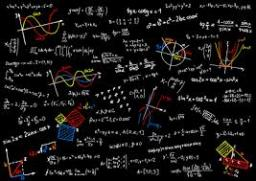# Properties: 2736

Find four numbers whose sum is 48 and which have the following properties: if we subtract 3 from the first, add 3 to the second, multiply the third by three, and divide the fourth by three, we get the same result.

a =  12
b =  6
c =  3
d =  27

### Step-by-step explanation:

a+b+c+d=48
a-3 = b+3
b+3 = 3c
3c = d/3

a+b+c+d=48
a-3 = b+3
b+3 = 3·c
3·c = d/3

a+b+c+d = 48
a-b = 6
b-3c = -3
9c-d = 0

Row 2 - Row 1 → Row 2
a+b+c+d = 48
-2b-c-d = -42
b-3c = -3
9c-d = 0

Row 3 - 1/-2 · Row 2 → Row 3
a+b+c+d = 48
-2b-c-d = -42
-3.5c-0.5d = -24
9c-d = 0

Pivot: Row 3 ↔ Row 4
a+b+c+d = 48
-2b-c-d = -42
9c-d = 0
-3.5c-0.5d = -24

Row 4 - -3.5/9 · Row 3 → Row 4
a+b+c+d = 48
-2b-c-d = -42
9c-d = 0
-0.8889d = -24

d = -24/-0.88888889 = 27
c = 0+d/9 = 0+27/9 = 3
b = -42+c+d/-2 = -42+3+27/-2 = 6
a = 48-b-c-d = 48-6-3-27 = 12

a = 12
b = 6
c = 3
d = 27

Our linear equations calculator calculates it.Did you find an error or inaccuracy? Feel free to write us. Thank you!

Tips for related online calculators
Do you have a system of equations and looking for calculator system of linear equations?